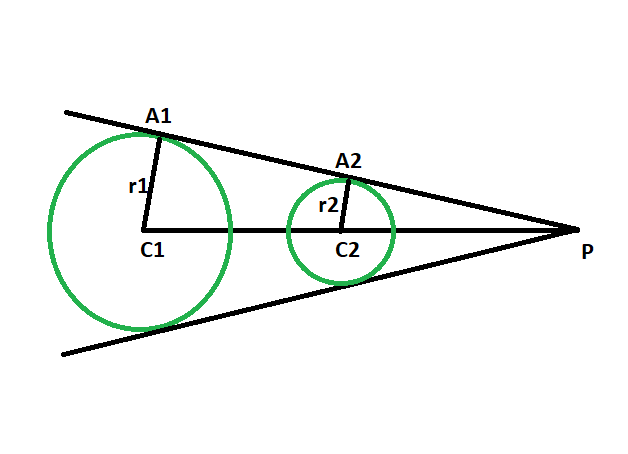GeeksforGeeks App
Open AppBrowser
Continue

# Ratio of the distance between the centers of the circles and the point of intersection of two direct common tangents to the circles

Given two circles of given radii, such that the circles don’t touch each other, the task is to find the ratio of the distance between the centers of the circles and the point of intersection of two direct common tangents to the circles.

Examples:

```Input: r1 = 4, r2 = 6
Output: 2:3

Input: r1 = 22, r2 = 43
Output: 22:43```Approach

• Let the radii of the circles be r1 & r2 and the centers be C1 & C2 respectively.
• Let P be the point of intersection of two direct common tangents to the circles, and A1 & A2 be the point of contact of the tangents with the circles.
• In triangle PC1A1 & triangle PC2A2
angle C1A1P = angle C2A2P = 90 deg { line joining the center of the circle to the point of contact makes an angle of 90 degree with the tangent },
also, angle A1PC1 = angle A2PC2
so, angle A1C1P = angle A2C2P
as angles are same, triangles PC1A1 & PC2A2 are similar.
• So, due to similarity of the triangles,
C1P/C2P = C1A1/C2A2 = r1/r2

The ratio of the distance between the centers of the circles and the point of intersection of two direct common tangents to the circles = radius of the first circle : radius of the second circle

Below is the implementation of the above approach:

## C++

 `// C++ program to find the ratio of the distance``// between the centers of the circles``// and the point of intersection of``// two direct common tangents to the circles``// which do not touch each other` `#include ``using` `namespace` `std;` `// Function to find the GCD``int` `GCD(``int` `a, ``int` `b)``{``    ``return` `(b != 0 ? GCD(b, a % b) : a);``}` `// Function to find the ratio``void` `ratiotang(``int` `r1, ``int` `r2)``{``    ``cout << ``"The ratio is "``         ``<< r1 / GCD(r1, r2)``         ``<< ``" : "``         ``<< r2 / GCD(r1, r2)``         ``<< endl;``}` `// Driver code``int` `main()``{``    ``int` `r1 = 4, r2 = 6;``    ``ratiotang(r1, r2);``    ``return` `0;``}`

## Java

 `// Java program to find the ratio of the distance``// between the centers of the circles``// and the point of intersection of``// two direct common tangents to the circles``// which do not touch each other``class` `GFG {` `    ``// Function to find the GCD``    ``static` `int` `GCD(``int` `a, ``int` `b)``    ``{``        ``return` `(b != ``0` `? GCD(b, a % b) : a);``    ``}` `    ``// Function to find the ratio``    ``static` `void` `ratiotang(``int` `r1, ``int` `r2)``    ``{``        ``System.out.println(``"The ratio is "``                           ``+ r1 / GCD(r1, r2)``                           ``+ ``" : "``                           ``+ r2 / GCD(r1, r2));``    ``}` `    ``// Driver code``    ``public` `static` `void` `main(String args[])``    ``{``        ``int` `r1 = ``4``, r2 = ``6``;``        ``ratiotang(r1, r2);``    ``}``}` `// This code has been contributed by 29AjayKumar`

## Python3

 `# Python 3 program to find the ratio``# of the distance between the centers``# of the circles and the point of intersection``# of two direct common tangents to the circles``# which do not touch each other` `# Function to find the GCD``from` `math ``import` `gcd` `# Function to find the ratio``def` `ratiotang(r1, r2):``    ``print``(``"The ratio is"``, ``int``(r1 ``/` `gcd(r1, r2)), ``":"``,``                          ``int``(r2 ``/` `gcd(r1, r2)))` `# Driver code``if` `__name__ ``=``=` `'__main__'``:``    ``r1 ``=` `4``    ``r2 ``=` `6``    ``ratiotang(r1, r2)` `# This code is contributed by``# Surendra_Gangwar`

## C#

 `// C# program to find the ratio of the distance``// between the centers of the circles``// and the point of intersection of``// two direct common tangents to the circles``// which do not touch each other``using` `System;``    ` `class` `GFG``{` `    ``// Function to find the GCD``    ``static` `int` `GCD(``int` `a, ``int` `b)``    ``{``        ``return` `(b != 0 ? GCD(b, a % b) : a);``    ``}` `    ``// Function to find the ratio``    ``static` `void` `ratiotang(``int` `r1, ``int` `r2)``    ``{``        ``Console.WriteLine(``"The ratio is "``                        ``+ r1 / GCD(r1, r2)``                        ``+ ``" : "``                        ``+ r2 / GCD(r1, r2));``    ``}` `    ``// Driver code``    ``public` `static` `void` `Main(String[] args)``    ``{``        ``int` `r1 = 4, r2 = 6;``        ``ratiotang(r1, r2);``    ``}``}` `// This code contributed by Rajput-Ji`

## PHP

 ``

## Javascript

 ``

Output:

`The ratio is 2 : 3`

Time Complexity: O(log(min(a, b)))

Auxiliary Space: O(log(min(a, b)))

My Personal Notes arrow_drop_up